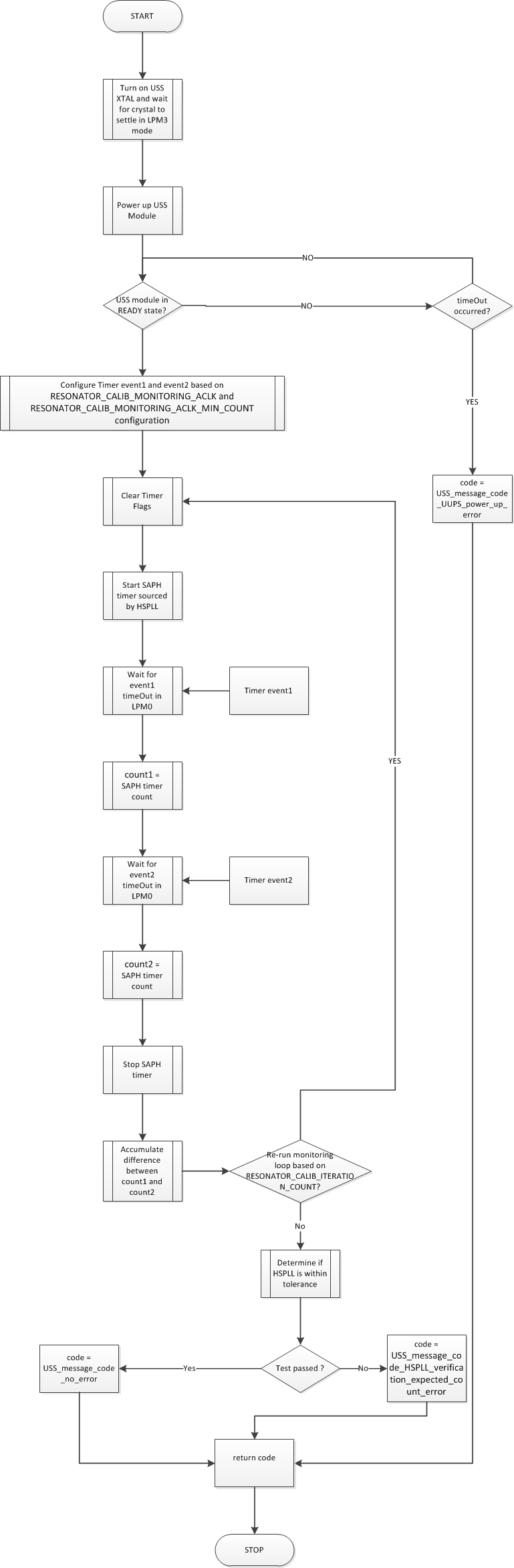# Library Calibrations Routines¶

## HSPLL Frequency Verification Test Configuration¶

The library allows user’s to compensate Absolute Time of Flight (AbsToF) and Delta Time of Flight (DToF) calculation due to USS XTAL frequency drift via `USS_Algorithms_User_Configuration.clockRelativeError` parameter.

It is highly recommended to use `USS_verifyHSPLLFrequency` API to configure `USS_Algorithms_User_Configuration.clockRelativeError`. The flow chart below shows the implementation for USS_verifyHSPLLFrequency API.Fig. 8 Clock Calibration

The default configuration parameters for this test are shown below. User’s can modify these parameters to increase test accuracy or reduce test duration. These parameters are available in <USS_LIB_DIR>/lib/USS/source/ussSwLibCalibration.h

```// Number of iterations used for averaging = 2^(RESONATOR_CALIB_ITERATION_COUNT)
// If RESONATOR_CALIB_ITERATION_COUNT == 0, Number of iterations used for
// averaging = 1.
// If RESONATOR_CALIB_ITERATION_COUNT == 1, Number of iterations used for
// averaging = 2
//
// Minimum value = 0
// Maximum value = 10
#define RESONATOR_CALIB_ITERATION_COUNT             2

// Number of ACLK cycles to monitor SAPH ASQCLK.
// Minimum value = 1
// Maximum value = 3000
#define RESONATOR_CALIB_MONITORING_ACLK             550
```

### Usage assumption¶

IMPORTANT:

During HSPLL frequency monitoring the application must ensure that no application level interrupts are triggered during the monitoring window otherwise HSPLL frequency calculation accuracy might be impacted.

### HSPLL Frequency Verification Test Benchmark¶

The table below shows the expected error bound, typical current consumption and test duration for various test configurations. Test duration were obtained using CCSv8.0 using optimization level -O3. Reducing the application optimization level will result in a longer test duration.

Configuration MCLK=8 MHz MCLK=16 MHz
Error bound Typical current consumption (μA) Typical Test duration (ms) Error bound Typical current consumption (μA) Typical test duration(ms)
``` RESONATOR_CALIB_ITERATION_COUNT == 2 RESONATOR_CALIB_MONITORING_ACLK == 550 ``` <1.86e-5 26.05 68.18 <9.31e-6 31.39 67.84
``` RESONATOR_CALIB_ITERATION_COUNT == 0 RESONATOR_CALIB_MONITORING_ACLK == 550 ``` <7.45e-5 7.01 17.41 <3.72e-5 8.41 17.19